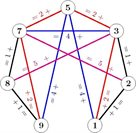# New mathematical discovery in maximal sum-free sets after a decade of the unknown

The College of Engineering and Physical Science Best Publication award September 2014 was awarded to Andrew Treglown, University of Birmingham and József Balogh, Hong Liu, Maryam Sharifzadeh, University of Illinois for ‘The number of maximal sum-free subsets of integers’, Proceedings of the American Mathematical Society, in press.

### Summary by Dr Andrew TreglownOne of the most basic concepts in Mathematics is the notion of a sum. That is, a set of three numbers such that two of the numbers add up to the third. For example, 4, 5 and 9 form the sum 4+5=9 whilst 3, 4 and 9 certainly do not form a sum! This concept is one that every school child has seen, yet there still remain many deep and difficult problems in this area.

We say that a set of numbers is sum-free if there are no three numbers in this set that form a sum. For example, if one considers any set of odd numbers then it is necessarily sum-free (since two odd numbers always add up to an even number). For decades many mathematicians have been fascinated by sum-free sets. In particular, they have been drawn to the question of what these sets ‘look like’ and, in a given collection of numbers, how many sum-free sets are there.

Indeed, in 1990 Cameron and Erdős posed a famous conjecture that asks for the number of sum-free sets in the set of the first n (natural) numbers 1, 2,..., n. This conjecture spawned a flurry of activity on the topic, and eventually, in celebrated work, the conjecture was solved independently by Green and Sapozhenko in 2004.

Mathematicians are also interested in how sum-free sets in the natural numbers are distributed. In particular, a large proportion of sum-free sets lie in just a few ‘maximal’ sum-free sets. (Roughly speaking, given a sum-free set of numbers, we say it is maximal if, when adding a new number to it, it no longer remains sum-free.) This led Cameron and Erdős to raise the question of how many maximal sum-free sets there are in the set of the first n (natural) numbers 1, 2,…, n.

Progress on this question proved more difficult. Indeed, until recently the problem remained wide-open. This year, in collaboration with mathematicians from the University of Illinois, we answered this question.

Whilst very much a question arising from Number Theory , our approach transformed it into a problem in a completely different area of Mathematics know as Graph Theory. Graph Theory concerns the study of networks and, in particular, a graph is a collection of points (known as vertices) some of which are connected together by lines (known as edges). At first sight, it does not seem at all obvious that there should be any link between a problem arising in Number Theory and such networks. It is interesting and perhaps surprising, therefore, that we found a deep rooted connection between counting maximal sum-free sets of numbers and so-called ‘independent sets’ in graphs.

This work is part of an exciting wider development in Mathematics using Graph Theoretical techniques to explore other branches of Mathematics such as Algebra, Number Theory and Set Theory.